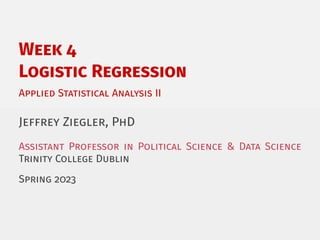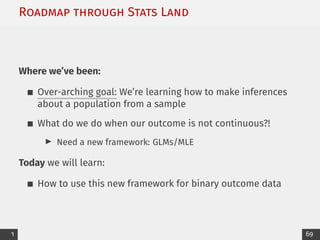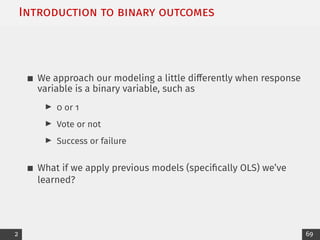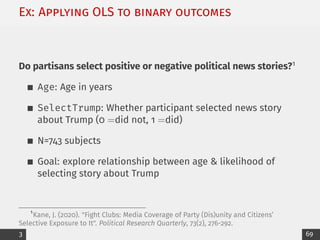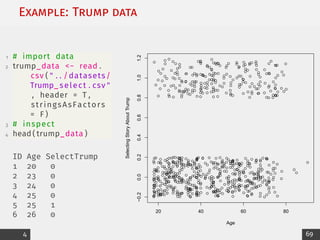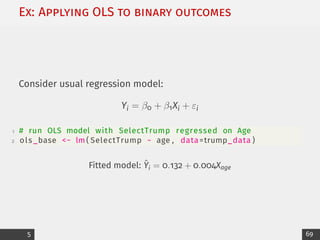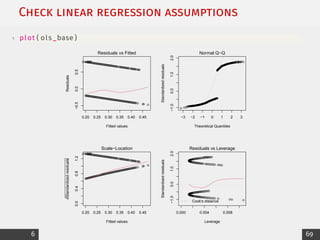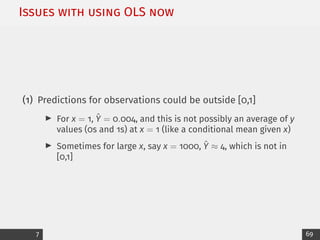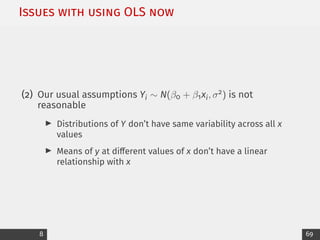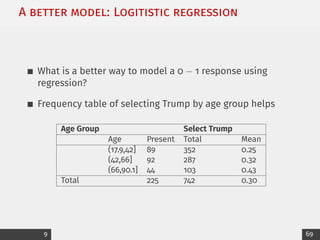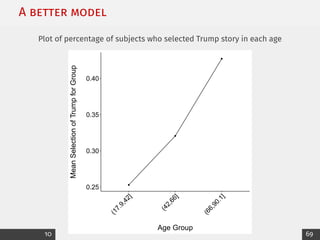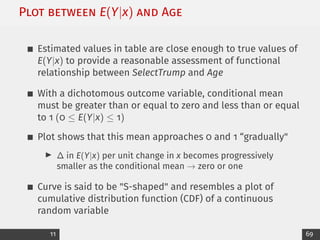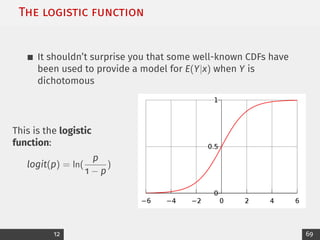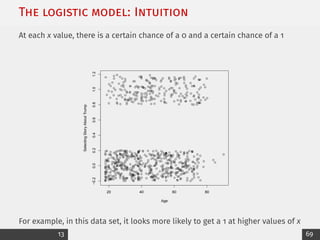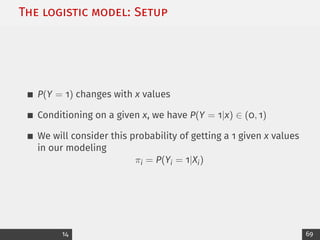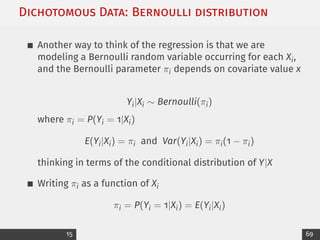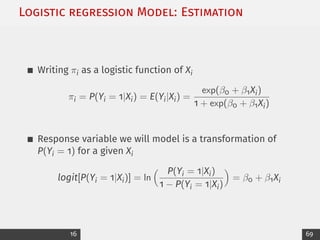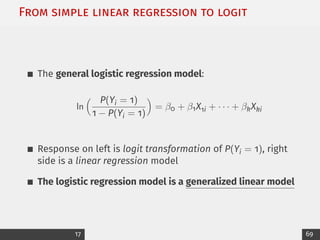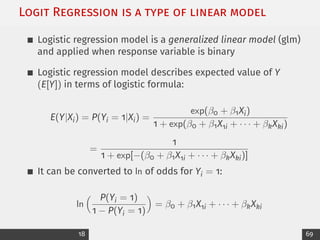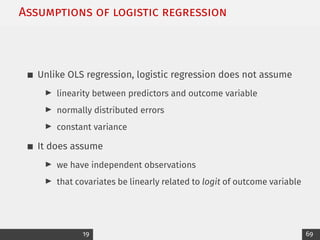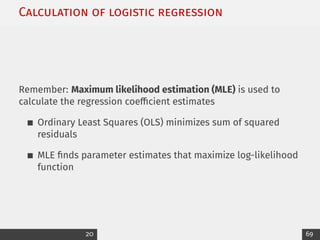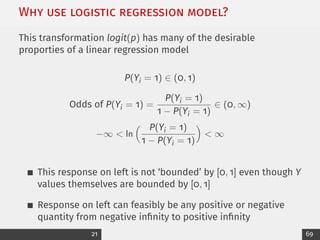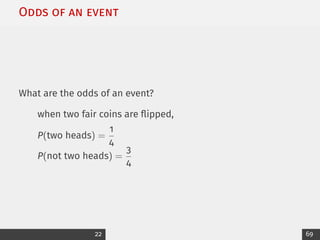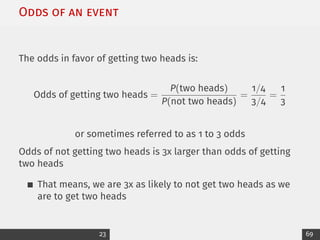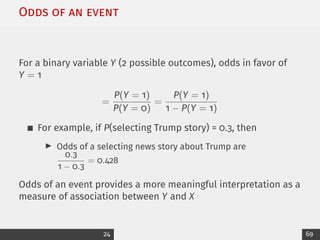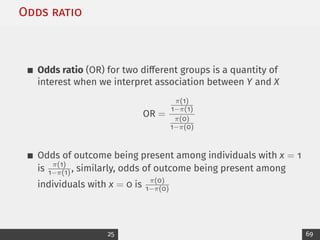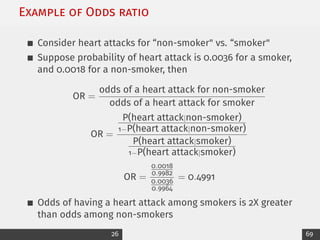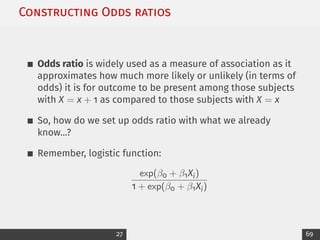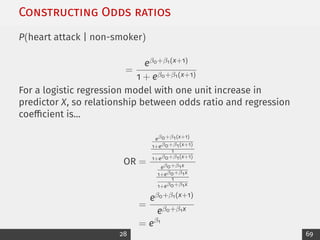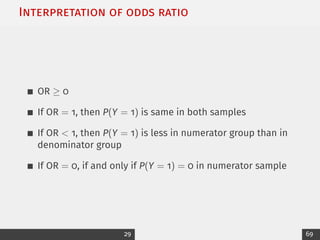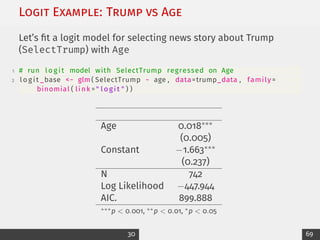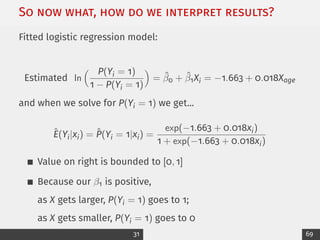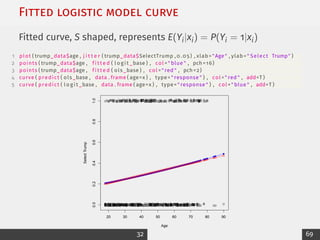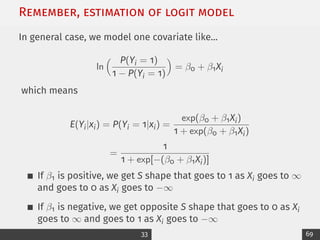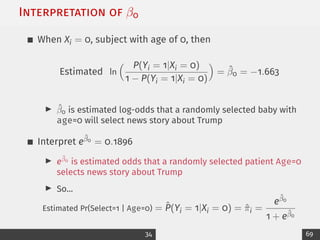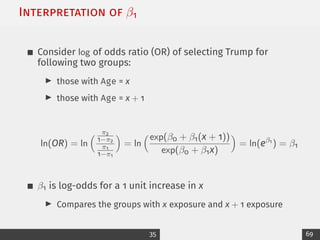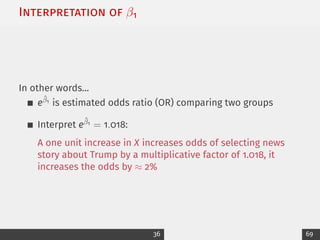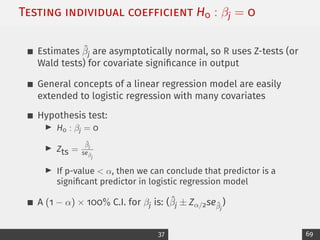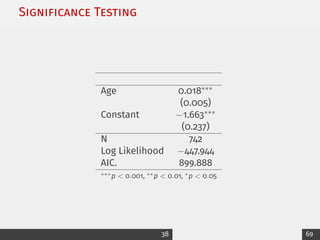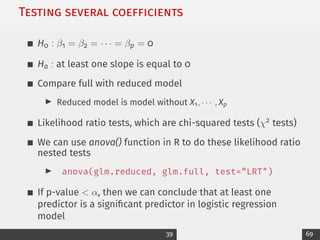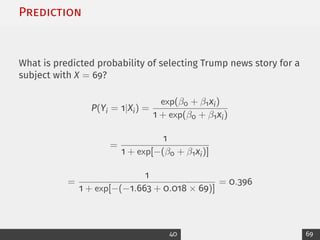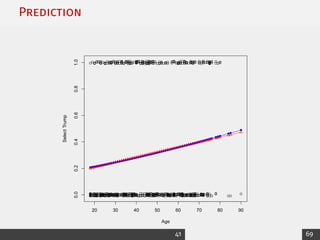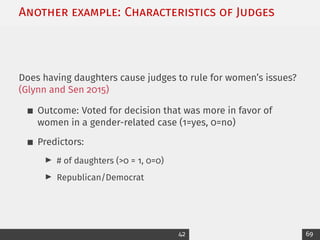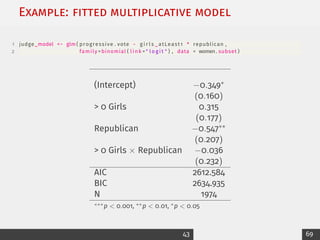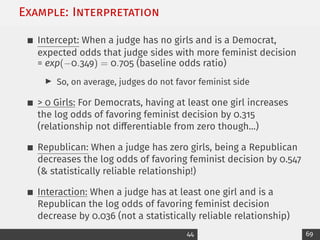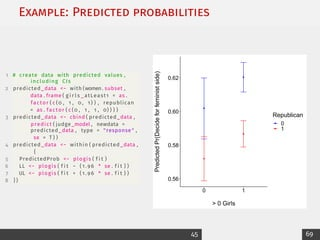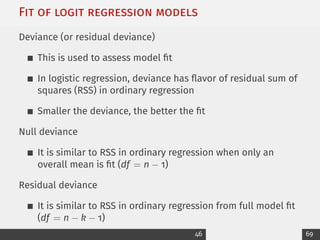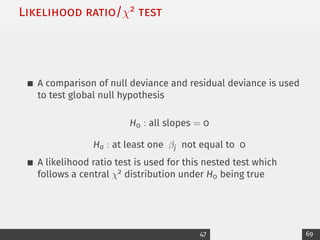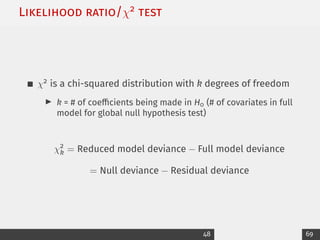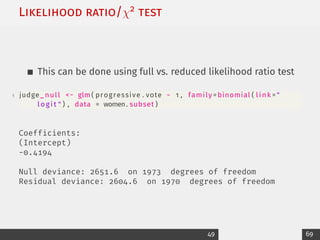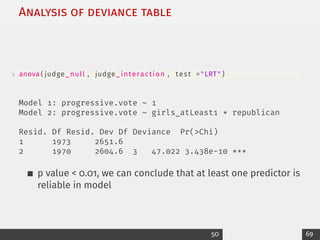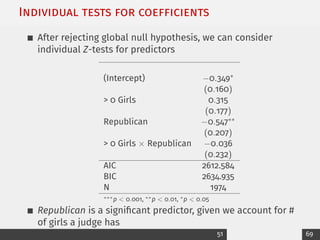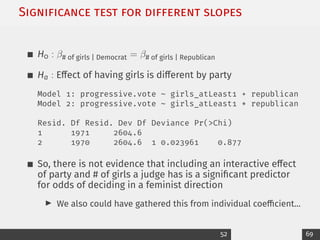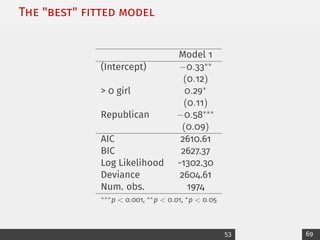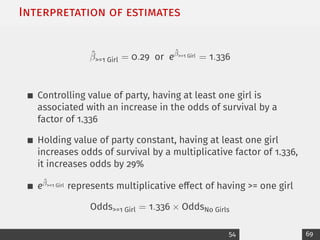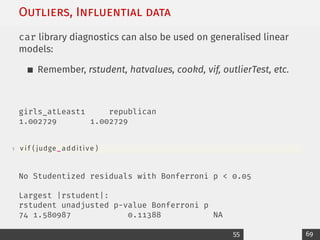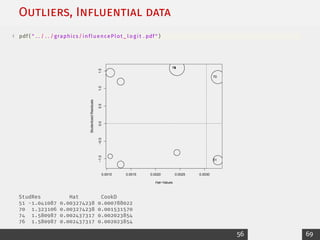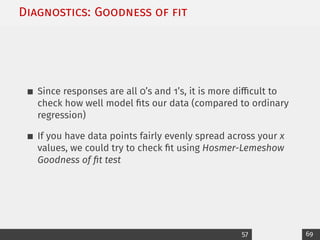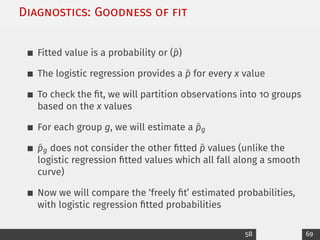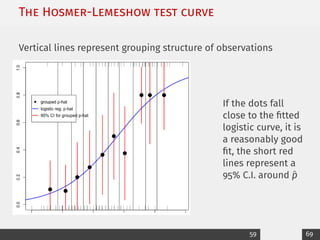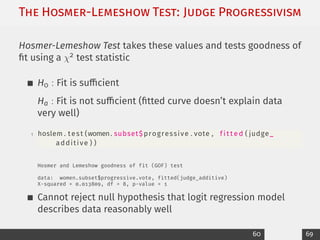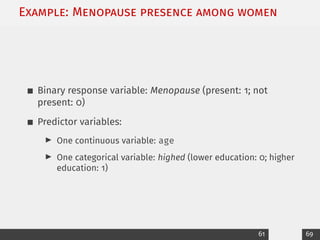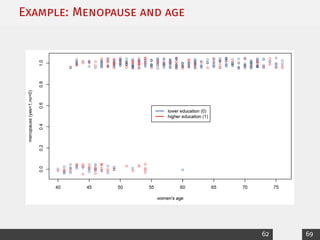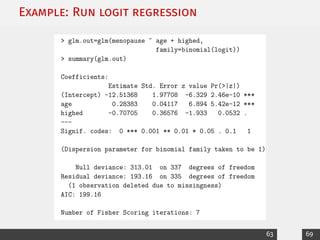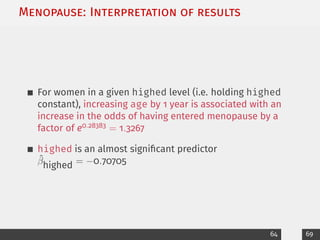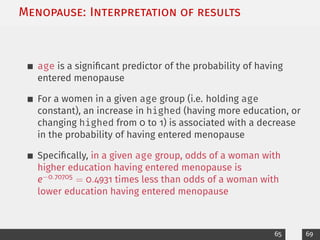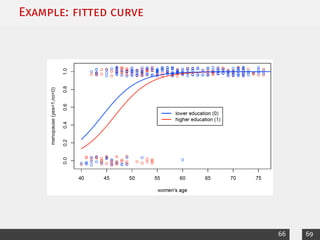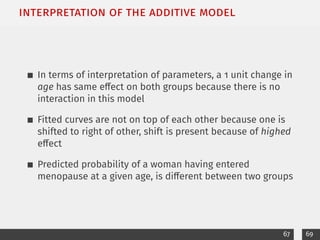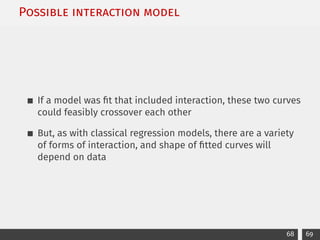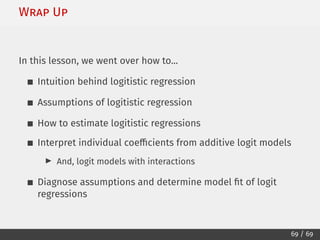1 de 70

### 4_logit_printable_.pdf

1. Week 4 Logistic Regression Applied Statistical Analysis II Jeffrey Ziegler, PhD Assistant Professor in Political Science & Data Science Trinity College Dublin Spring 2023
2. Roadmap through Stats Land Where we’ve been: Over-arching goal: We’re learning how to make inferences about a population from a sample What do we do when our outcome is not continuous?! I Need a new framework: GLMs/MLE Today we will learn: How to use this new framework for binary outcome data 1 69
3. Introduction to binary outcomes We approach our modeling a little differently when response variable is a binary variable, such as I 0 or 1 I Vote or not I Success or failure What if we apply previous models (specifically OLS) we’ve learned? 2 69
4. Ex: Applying OLS to binary outcomes Do partisans select positive or negative political news stories?1 Age: Age in years SelectTrump: Whether participant selected news story about Trump (0 =did not, 1 =did) N=743 subjects Goal: explore relationship between age & likelihood of selecting story about Trump 1 Kane, J. (2020). "Fight Clubs: Media Coverage of Party (Dis)unity and Citizens’ Selective Exposure to It". Political Research Quarterly, 73(2), 276-292. 3 69
5. Example: Trump data 1 # import data 2 trump_data <− read . csv ( " . . /datasets/ Trump_ select . csv " , header = T , stringsAsFactors = F ) 3 # inspect 4 head ( trump_data ) ID Age SelectTrump 1 20 0 2 23 0 3 24 0 4 25 0 5 25 1 6 26 0 20 40 60 80 −0.2 0.0 0.2 0.4 0.6 0.8 1.0 1.2 Age Selecting Story About Trump 4 69
6. Ex: Applying OLS to binary outcomes Consider usual regression model: Yi = β0 + β1Xi + εi 1 # run OLS model with SelectTrump regressed on Age 2 ols _base <− lm ( SelectTrump ~ age , data=trump_data ) Fitted model: Ŷi = 0.132 + 0.004Xage 5 69
7. Check linear regression assumptions 1 plot ( ols _base ) 0.20 0.25 0.30 0.35 0.40 0.45 −0.5 0.0 0.5 Fitted values Residuals Residuals vs Fitted 549 675 239 −3 −2 −1 0 1 2 3 −1.0 0.0 1.0 2.0 Theoretical Quantiles Standardized residuals Normal Q−Q 549 675 239 0.20 0.25 0.30 0.35 0.40 0.45 0.0 0.4 0.8 1.2 Fitted values Standardized residuals Scale−Location 549 675 239 0.000 0.004 0.008 −1.0 0.0 1.0 2.0 Leverage Standardized residuals Cook's distance Residuals vs Leverage 549 675 239 6 69
8. Issues with using OLS now (1) Predictions for observations could be outside [0,1] I For x = 1, Ŷ = 0.004, and this is not possibly an average of y values (0s and 1s) at x = 1 (like a conditional mean given x) I Sometimes for large x, say x = 1000, Ŷ ≈ 4, which is not in [0,1] 7 69
9. Issues with using OLS now (2) Our usual assumptions Yi ∼ N(β0 + β1xi, σ2) is not reasonable I Distributions of Y don’t have same variability across all x values I Means of y at different values of x don’t have a linear relationship with x 8 69
10. A better model: Logitistic regression What is a better way to model a 0 − 1 response using regression? Frequency table of selecting Trump by age group helps Age Group Select Trump Age Present Total Mean (17.9,42] 89 352 0.25 (42,66] 92 287 0.32 (66,90.1] 44 103 0.43 Total 225 742 0.30 9 69
11. A better model Plot of percentage of subjects who selected Trump story in each age 0.25 0.30 0.35 0.40 ( 1 7 . 9 , 4 2 ] ( 4 2 , 6 6 ] ( 6 6 , 9 0 . 1 ] Age Group Mean Selection of Trump for Group 10 69
12. Plot between E(Y|x) and Age Estimated values in table are close enough to true values of E(Y|x) to provide a reasonable assessment of functional relationship between SelectTrump and Age With a dichotomous outcome variable, conditional mean must be greater than or equal to zero and less than or equal to 1 (0 ≤ E(Y|x) ≤ 1) Plot shows that this mean approaches 0 and 1 “gradually" I ∆ in E(Y|x) per unit change in x becomes progressively smaller as the conditional mean → zero or one Curve is said to be "S-shaped" and resembles a plot of cumulative distribution function (CDF) of a continuous random variable 11 69
13. The logistic function It shouldn’t surprise you that some well-known CDFs have been used to provide a model for E(Y|x) when Y is dichotomous This is the logistic function: logit(p) = ln( p 1 − p ) 12 69
14. The logistic model: Intuition At each x value, there is a certain chance of a 0 and a certain chance of a 1 20 40 60 80 −0.2 0.0 0.2 0.4 0.6 0.8 1.0 1.2 Age Selecting Story About Trump For example, in this data set, it looks more likely to get a 1 at higher values of x 13 69
15. The logistic model: Setup P(Y = 1) changes with x values Conditioning on a given x, we have P(Y = 1|x) ∈ (0, 1) We will consider this probability of getting a 1 given x values in our modeling πi = P(Yi = 1|Xi) 14 69
16. Dichotomous Data: Bernoulli distribution Another way to think of the regression is that we are modeling a Bernoulli random variable occurring for each Xi, and the Bernoulli parameter πi depends on covariate value x Yi|Xi ∼ Bernoulli(πi) where πi = P(Yi = 1|Xi) E(Yi|Xi) = πi and Var(Yi|Xi) = πi(1 − πi) thinking in terms of the conditional distribution of Y|X Writing πi as a function of Xi πi = P(Yi = 1|Xi) = E(Yi|Xi) 15 69
17. Logistic regression Model: Estimation Writing πi as a logistic function of Xi πi = P(Yi = 1|Xi) = E(Yi|Xi) = exp(β0 + β1Xi) 1 + exp(β0 + β1Xi) Response variable we will model is a transformation of P(Yi = 1) for a given Xi logit[P(Yi = 1|Xi)] = ln P(Yi = 1|Xi) 1 − P(Yi = 1|Xi) = β0 + β1Xi 16 69
18. From simple linear regression to logit The general logistic regression model: ln P(Yi = 1) 1 − P(Yi = 1) = β0 + β1X1i + · · · + βkXki Response on left is logit transformation of P(Yi = 1), right side is a linear regression model The logistic regression model is a generalized linear model 17 69
19. Logit Regression is a type of linear model Logistic regression model is a generalized linear model (glm) and applied when response variable is binary Logistic regression model describes expected value of Y (E[Y]) in terms of logistic formula: E(Y|Xi) = P(Yi = 1|Xi) = exp(β0 + β1Xi) 1 + exp(β0 + β1X1i + · · · + βkXki) = 1 1 + exp[−(β0 + β1X1i + · · · + βkXki)] It can be converted to ln of odds for Yi = 1: ln P(Yi = 1) 1 − P(Yi = 1) = β0 + β1X1i + · · · + βkXki 18 69
20. Assumptions of logistic regression Unlike OLS regression, logistic regression does not assume I linearity between predictors and outcome variable I normally distributed errors I constant variance It does assume I we have independent observations I that covariates be linearly related to logit of outcome variable 19 69
21. Calculation of logistic regression Remember: Maximum likelihood estimation (MLE) is used to calculate the regression coefficient estimates Ordinary Least Squares (OLS) minimizes sum of squared residuals MLE finds parameter estimates that maximize log-likelihood function 20 69
22. Why use logistic regression model? This transformation logit(p) has many of the desirable proporties of a linear regression model P(Yi = 1) ∈ (0, 1) Odds of P(Yi = 1) = P(Yi = 1) 1 − P(Yi = 1) ∈ (0, ∞) −∞ ln P(Yi = 1) 1 − P(Yi = 1) ∞ This response on left is not ‘bounded’ by [0, 1] even though Y values themselves are bounded by [0, 1] Response on left can feasibly be any positive or negative quantity from negative infinity to positive infinity 21 69
23. Odds of an event What are the odds of an event? when two fair coins are flipped, P(two heads) = 1 4 P(not two heads) = 3 4 22 69
24. Odds of an event The odds in favor of getting two heads is: Odds of getting two heads = P(two heads) P(not two heads) = 1/4 3/4 = 1 3 or sometimes referred to as 1 to 3 odds Odds of not getting two heads is 3x larger than odds of getting two heads That means, we are 3x as likely to not get two heads as we are to get two heads 23 69
25. Odds of an event For a binary variable Y (2 possible outcomes), odds in favor of Y = 1 = P(Y = 1) P(Y = 0) = P(Y = 1) 1 − P(Y = 1) For example, if P(selecting Trump story) = 0.3, then I Odds of a selecting news story about Trump are 0.3 1 − 0.3 = 0.428 Odds of an event provides a more meaningful interpretation as a measure of association between Y and X 24 69
26. Odds ratio Odds ratio (OR) for two different groups is a quantity of interest when we interpret association between Y and X OR = π(1) 1−π(1) π(0) 1−π(0) Odds of outcome being present among individuals with x = 1 is π(1) 1−π(1) , similarly, odds of outcome being present among individuals with x = 0 is π(0) 1−π(0) 25 69
27. Example of Odds ratio Consider heart attacks for “non-smoker vs. “smoker Suppose probability of heart attack is 0.0036 for a smoker, and 0.0018 for a non-smoker, then OR = odds of a heart attack for non-smoker odds of a heart attack for smoker OR = P(heart attack|non-smoker) 1−P(heart attack|non-smoker) P(heart attack|smoker) 1−P(heart attack|smoker) OR = 0.0018 0.9982 0.0036 0.9964 = 0.4991 Odds of having a heart attack among smokers is 2X greater than odds among non-smokers 26 69
28. Constructing Odds ratios Odds ratio is widely used as a measure of association as it approximates how much more likely or unlikely (in terms of odds) it is for outcome to be present among those subjects with X = x + 1 as compared to those subjects with X = x So, how do we set up odds ratio with what we already know...? Remember, logistic function: exp(β0 + β1Xi) 1 + exp(β0 + β1Xi) 27 69
29. Constructing Odds ratios P(heart attack | non-smoker) = eβ0+β1(x+1) 1 + eβ0+β1(x+1) For a logistic regression model with one unit increase in predictor X, so relationship between odds ratio and regression coefficient is... OR = eβ0+β1(x+1) 1+eβ0+β1(x+1) 1 1+eβ0+β1(x+1) eβ0+β1x 1+eβ0+β1x 1 1+eβ0+β1x = eβ0+β1(x+1) eβ0+β1x = eβ1 28 69
30. Interpretation of odds ratio OR ≥ 0 If OR = 1, then P(Y = 1) is same in both samples If OR 1, then P(Y = 1) is less in numerator group than in denominator group If OR = 0, if and only if P(Y = 1) = 0 in numerator sample 29 69
31. Logit Example: Trump vs Age Let’s fit a logit model for selecting news story about Trump (SelectTrump) with Age 1 # run l o g i t model with SelectTrump regressed on Age 2 l o g i t _base − glm ( SelectTrump ~ age , data=trump_data , family = binomial ( link = l o g i t ) ) Age 0.018∗∗∗ (0.005) Constant −1.663∗∗∗ (0.237) N 742 Log Likelihood −447.944 AIC. 899.888 ∗∗∗p 0.001, ∗∗p 0.01, ∗p 0.05 30 69
32. So now what, how do we interpret results? Fitted logistic regression model: Estimated ln P(Yi = 1) 1 − P(Yi = 1) = β̂0 + β̂1Xi = −1.663 + 0.018Xage and when we solve for P(Yi = 1) we get... Ê(Yi|xi) = P̂(Yi = 1|xi) = exp(−1.663 + 0.018xi) 1 + exp(−1.663 + 0.018xi) Value on right is bounded to [0, 1] Because our β1 is positive, as X gets larger, P(Yi = 1) goes to 1; as X gets smaller, P(Yi = 1) goes to 0 31 69
33. Fitted logistic model curve Fitted curve, S shaped, represents E(Yi|xi) = P(Yi = 1|xi) 1 plot ( trump_data\$age , j i t t e r ( trump_data\$SelectTrump , 0 . 0 5 ) , xlab=Age , ylab= Select Trump ) 2 points ( trump_data\$age , f i t t e d ( l o g i t _base ) , col = blue , pch =16) 3 points ( trump_data\$age , f i t t e d ( ols _base ) , col = red , pch =2) 4 curve ( predict ( ols _base , data . frame ( age=x ) , type= response ) , col = red , add=T ) 5 curve ( predict ( l o g i t _base , data . frame ( age=x ) , type= response ) , col = blue , add=T ) 20 30 40 50 60 70 80 90 0.0 0.2 0.4 0.6 0.8 1.0 Age Select Trump 32 69
34. Remember, estimation of logit model In general case, we model one covariate like... ln P(Yi = 1) 1 − P(Yi = 1) = β0 + β1Xi which means E(Yi|xi) = P(Yi = 1|xi) = exp(β0 + β1Xi) 1 + exp(β0 + β1Xi) = 1 1 + exp[−(β0 + β1Xi)] If β1 is positive, we get S shape that goes to 1 as Xi goes to ∞ and goes to 0 as Xi goes to −∞ If β1 is negative, we get opposite S shape that goes to 0 as Xi goes to ∞ and goes to 1 as Xi goes to −∞ 33 69
35. Interpretation of β0 When Xi = 0, subject with age of 0, then Estimated ln P(Yi = 1|Xi = 0) 1 − P(Yi = 1|Xi = 0) = β̂0 = −1.663 I β̂0 is estimated log-odds that a randomly selected baby with age=0 will select news story about Trump Interpret eβ̂0 = 0.1896 I eβ̂0 is estimated odds that a randomly selected patient Age=0 selects news story about Trump I So... Estimated Pr(Select=1 | Age=0) = P̂(Yi = 1|Xi = 0) = π̂i = eβ̂0 1 + eβ̂0 34 69
36. Interpretation of β1 Consider log of odds ratio (OR) of selecting Trump for following two groups: I those with Age = x I those with Age = x + 1 ln(OR) = ln π2 1−π2 π1 1−π1 = ln exp(β0 + β1(x + 1)) exp(β0 + β1x) = ln(eβ1 ) = β1 β1 is log-odds for a 1 unit increase in x I Compares the groups with x exposure and x + 1 exposure 35 69
37. Interpretation of β1 In other words... eβ̂1 is estimated odds ratio (OR) comparing two groups Interpret eβ̂1 = 1.018: A one unit increase in X increases odds of selecting news story about Trump by a multiplicative factor of 1.018, it increases the odds by ≈ 2% 36 69
38. Testing individual coefﬁcient H0 : βj = 0 Estimates β̂j are asymptotically normal, so R uses Z-tests (or Wald tests) for covariate significance in output General concepts of a linear regression model are easily extended to logistic regression with many covariates Hypothesis test: I H0 : βj = 0 I Zts = β̂j seβ̂j I If p-value α, then we can conclude that predictor is a significant predictor in logistic regression model A (1 − α) × 100% C.I. for βj is: (β̂j ± Zα/2seβ̂j ) 37 69
39. Signiﬁcance Testing Age 0.018∗∗∗ (0.005) Constant −1.663∗∗∗ (0.237) N 742 Log Likelihood −447.944 AIC. 899.888 ∗∗∗p 0.001, ∗∗p 0.01, ∗p 0.05 38 69
40. Testing several coefﬁcients H0 : β1 = β2 = · · · = βp = 0 Ha : at least one slope is equal to 0 Compare full with reduced model I Reduced model is model without X1, · · · , Xp Likelihood ratio tests, which are chi-squared tests (χ2 tests) We can use anova() function in R to do these likelihood ratio nested tests I anova(glm.reduced, glm.full, test=“LRT) If p-value α, then we can conclude that at least one predictor is a significant predictor in logistic regression model 39 69
41. Prediction What is predicted probability of selecting Trump news story for a subject with X = 69? P(Yi = 1|Xi) = exp(β0 + β1xi) 1 + exp(β0 + β1xi) = 1 1 + exp[−(β0 + β1xi)] = 1 1 + exp[−(−1.663 + 0.018 × 69)] = 0.396 40 69
42. Prediction 20 30 40 50 60 70 80 90 0.0 0.2 0.4 0.6 0.8 1.0 Age Select Trump 41 69
43. Another example: Characteristics of Judges Does having daughters cause judges to rule for women’s issues? (Glynn and Sen 2015) Outcome: Voted for decision that was more in favor of women in a gender-related case (1=yes, 0=no) Predictors: I # of daughters (0 = 1, 0=0) I Republican/Democrat 42 69
44. Example: ﬁtted multiplicative model 1 judge_model − glm ( progressive . vote ~ g i r l s _ atLeast1 * republican , 2 family =binomial ( link = l o g i t ) , data = women. subset ) (Intercept) −0.349∗ (0.160) 0 Girls 0.315 (0.177) Republican −0.547∗∗ (0.207) 0 Girls × Republican −0.036 (0.232) AIC 2612.584 BIC 2634.935 N 1974 ∗∗∗p 0.001, ∗∗p 0.01, ∗p 0.05 43 69
45. Example: Interpretation Intercept: When a judge has no girls and is a Democrat, expected odds that judge sides with more feminist decision = exp(−0.349) = 0.705 (baseline odds ratio) I So, on average, judges do not favor feminist side 0 Girls: For Democrats, having at least one girl increases the log odds of favoring feminist decision by 0.315 (relationship not differentiable from zero though...) Republican: When a judge has zero girls, being a Republican decreases the log odds of favoring feminist decision by 0.547 ( statistically reliable relationship!) Interaction: When a judge has at least one girl and is a Republican the log odds of favoring feminist decision decrease by 0.036 (not a statistically reliable relationship) 44 69
46. Example: Predicted probabilities 1 # create data with predicted values , including CIs 2 predicted_data − with (women. subset , data . frame ( g i r l s _ atLeast1 = as . factor ( c (0 , 1 , 0 , 1 ) ) , republican = as . factor ( c (0 , 1 , 1 , 0) ) ) ) 3 predicted_data − cbind ( predicted_data , predict ( judge_model , newdata = predicted_data , type = response , se = T ) ) 4 predicted_data − within ( predicted_data , { 5 PredictedProb − plogis ( f i t ) 6 LL − plogis ( f i t − ( 1 . 9 6 * se . f i t ) ) 7 UL − plogis ( f i t + ( 1 . 9 6 * se . f i t ) ) 8 } ) ● ● ● ● 0.56 0.58 0.60 0.62 0 1 0 Girls Predicted Pr(Decide for feminist side) Republican ● ● 0 1 45 69
47. Fit of logit regression models Deviance (or residual deviance) This is used to assess model fit In logistic regression, deviance has flavor of residual sum of squares (RSS) in ordinary regression Smaller the deviance, the better the fit Null deviance It is similar to RSS in ordinary regression when only an overall mean is fit (df = n − 1) Residual deviance It is similar to RSS in ordinary regression from full model fit (df = n − k − 1) 46 69
48. Likelihood ratio/χ2 test A comparison of null deviance and residual deviance is used to test global null hypothesis H0 : all slopes = 0 Ha : at least one βj not equal to 0 A likelihood ratio test is used for this nested test which follows a central χ2 distribution under H0 being true 47 69
49. Likelihood ratio/χ2 test χ2 is a chi-squared distribution with k degrees of freedom I k = # of coefficients being made in H0 (# of covariates in full model for global null hypothesis test) χ2 k = Reduced model deviance − Full model deviance = Null deviance − Residual deviance 48 69
50. Likelihood ratio/χ2 test This can be done using full vs. reduced likelihood ratio test 1 judge_ null − glm ( progressive . vote ~ 1 , family =binomial ( link = l o g i t ) , data = women. subset ) Coefficients: (Intercept) -0.4194 Null deviance: 2651.6 on 1973 degrees of freedom Residual deviance: 2604.6 on 1970 degrees of freedom 49 69
51. Analysis of deviance table 1 anova ( judge_null , judge_ interaction , test =LRT ) Model 1: progressive.vote ~ 1 Model 2: progressive.vote ~ girls_atLeast1 * republican Resid. Df Resid. Dev Df Deviance Pr(Chi) 1 1973 2651.6 2 1970 2604.6 3 47.022 3.438e-10 *** p value 0.01, we can conclude that at least one predictor is reliable in model 50 69
52. Individual tests for coefﬁcients After rejecting global null hypothesis, we can consider individual Z-tests for predictors (Intercept) −0.349∗ (0.160) 0 Girls 0.315 (0.177) Republican −0.547∗∗ (0.207) 0 Girls × Republican −0.036 (0.232) AIC 2612.584 BIC 2634.935 N 1974 ∗∗∗p 0.001, ∗∗p 0.01, ∗p 0.05 Republican is a significant predictor, given we account for # of girls a judge has 51 69
53. Signiﬁcance test for different slopes H0 : β# of girls | Democrat = β# of girls | Republican Ha : Effect of having girls is different by party Model 1: progressive.vote ~ girls_atLeast1 + republican Model 2: progressive.vote ~ girls_atLeast1 * republican Resid. Df Resid. Dev Df Deviance Pr(Chi) 1 1971 2604.6 2 1970 2604.6 1 0.023961 0.877 So, there is not evidence that including an interactive effect of party and # of girls a judge has is a significant predictor for odds of deciding in a feminist direction I We also could have gathered this from individual coefficient... 52 69
54. The best ﬁtted model Model 1 (Intercept) −0.33∗∗ (0.12) 0 girl 0.29∗ (0.11) Republican −0.58∗∗∗ (0.09) AIC 2610.61 BIC 2627.37 Log Likelihood -1302.30 Deviance 2604.61 Num. obs. 1974 ∗∗∗p 0.001, ∗∗p 0.01, ∗p 0.05 53 69
55. Interpretation of estimates β̂=1 Girl = 0.29 or eβ̂=1 Girl = 1.336 Controlling value of party, having at least one girl is associated with an increase in the odds of survival by a factor of 1.336 Holding value of party constant, having at least one girl increases odds of survival by a multiplicative factor of 1.336, it increases odds by 29% eβ̂=1 Girl represents multiplicative effect of having = one girl Odds=1 Girl = 1.336 × OddsNo Girls 54 69
56. Outliers, Inﬂuential data car library diagnostics can also be used on generalised linear models: Remember, rstudent, hatvalues, cookd, vif, outlierTest, etc. girls_atLeast1 republican 1.002729 1.002729 1 v i f ( judge_ additive ) No Studentized residuals with Bonferroni p 0.05 Largest |rstudent|: rstudent unadjusted p-value Bonferroni p 74 1.580987 0.11388 NA 55 69
57. Outliers, Inﬂuential data 1 pdf ( . . / . . /graphics/ influencePlot _ l o g i t . pdf ) 0.0010 0.0015 0.0020 0.0025 0.0030 −1.0 −0.5 0.0 0.5 1.0 1.5 Hat−Values Studentized Residuals ● ● ● ● ● ● ● ● ● ● ● ● ● ● ● ● ● ● ● ● ● ● ● ● ● ● ● ● ● ● ● ● ● ● ● ● ● ● ● ● ● ● ● ● ● ● ● ● ● ● ● ● ● ● ● ● ● ● ● ● ● ● ● ● ● ● ● ● ● ● ● ● ● ● ● ● ● ● ● ● ● ● ● ● ● ● ● ● ● ● ● ● ● ● ● ● ● ● ● ● ● ● ● ● ● ● ● ● ● ● ● ● ● ● ● ● ● ● ● ● ● ● ● ● ● ● ● ● ● ● ● ● ● ● ● ● ● ● ● ● ● ● ● ● ● ● ● ● ● ● ● ● ● ● ● ● ● ● ● ● ● ● ● ● ● ● ● ● ● ● ● ● ● ● ● ● ● ● ● ● ● ● ● ● ● ● ● ● ● ● ● ● ● ● ● ● ● ● ● ● ● ● ● ● ● ● ● ● ● ● ● ● ● ● ● ● ● ● ● ● ● ● ● ● ● ● ● ● ● ● ● ● ● ● ● ● ● ● ● ● ● ● ● ● ● ● ● ● ● ● ● ● ● ● ● ● ● ● ● ● ● ● ● ● ● ● ● ● ● ● ● ● ● ● ● ● ● ● ● ● ● ● ● ● ● ● ● ● ● ● ● ● ● ● ● ● ● ● ● ● ● ● ● ● ● ● ● ● ● ● ● ● ● ● ● ● ● ● ● ● ● ● ● ● ● ● ● ● ● ● ● ● ● ● ● ● ● ● ● ● ● ● ● ● ● ● ● ● ● ● ● ● ● ● ● ● ● ● ● ● ● ● ● ● ● ● ● ● ● ● ● ● ● ● ● ● ● ● ● ● ● ● ● ● ● ● ● ● ● ● ● ● ● ● ● ● ● ● ● ● ● ● ● ● ● ● ● ● ● ● ● ● ● ● ● ● ● ● ● ● ● ● ● ● ● ● ● ● ● ● ● ● ● ● ● ● ● ● ● ● ● ● ● ● ● ● ● ● ● ● ● ● ● ● ● ● ● ● ● ● ● ● ● ● ● ● ● ● ● ● ● ● ● ● ● ● ● ● ● ● ● ● ● ● ● ● ● ● ● ● ● ● ● ● ● ● ● ● ● ● ● ● ● ● ● ● ● ● ● ● ● ● ● ● ● ● ● ● ● ● ● ● ● ● ● ● ● ● ● ● ● ● ● ● ● ● ● ● ● ● ● ● ● ● ● ● ● 51 70 74 76 StudRes Hat CookD 51 -1.041087 0.003274238 0.000788022 70 1.323106 0.003274238 0.001531570 74 1.580987 0.002437317 0.002023854 76 1.580987 0.002437317 0.002023854 56 69
58. Diagnostics: Goodness of ﬁt Since responses are all 0’s and 1’s, it is more difficult to check how well model fits our data (compared to ordinary regression) If you have data points fairly evenly spread across your x values, we could try to check fit using Hosmer-Lemeshow Goodness of fit test 57 69
59. Diagnostics: Goodness of ﬁt Fitted value is a probability or (p̂) The logistic regression provides a p̂ for every x value To check the fit, we will partition observations into 10 groups based on the x values For each group g, we will estimate a p̂g p̂g does not consider the other fitted p̂ values (unlike the logistic regression fitted values which all fall along a smooth curve) Now we will compare the ‘freely fit’ estimated probabilities, with logistic regression fitted probabilities 58 69
60. The Hosmer-Lemeshow test curve Vertical lines represent grouping structure of observations If the dots fall close to the fitted logistic curve, it is a reasonably good fit, the short red lines represent a 95% C.I. around p̂ 59 69
61. The Hosmer-Lemeshow Test: Judge Progressivism Hosmer-Lemeshow Test takes these values and tests goodness of fit using a χ2 test statistic H0 : Fit is sufficient Ha : Fit is not sufficient (fitted curve doesn’t explain data very well) 1 hoslem . test (women. subset\$progressive . vote , f i t t e d ( judge_ additive ) ) Hosmer and Lemeshow goodness of fit (GOF) test data: women.subset\$progressive.vote, fitted(judge_additive) X-squared = 0.013809, df = 8, p-value = 1 Cannot reject null hypothesis that logit regression model describes data reasonably well 60 69
62. Example: Menopause presence among women Binary response variable: Menopause (present: 1; not present: 0) Predictor variables: I One continuous variable: age I One categorical variable: highed (lower education: 0; higher education: 1) 61 69
63. Example: Menopause and age 62 69
64. Example: Run logit regression 63 69
65. Menopause: Interpretation of results For women in a given highed level (i.e. holding highed constant), increasing age by 1 year is associated with an increase in the odds of having entered menopause by a factor of e0.28383 = 1.3267 highed is an almost significant predictor β̂highed = −0.70705 64 69
66. Menopause: Interpretation of results age is a significant predictor of the probability of having entered menopause For a women in a given age group (i.e. holding age constant), an increase in highed (having more education, or changing highed from 0 to 1) is associated with a decrease in the probability of having entered menopause Specifically, in a given age group, odds of a woman with higher education having entered menopause is e−0.70705 = 0.4931 times less than odds of a woman with lower education having entered menopause 65 69
67. Example: ﬁtted curve 66 69
68. interpretation of the additive model In terms of interpretation of parameters, a 1 unit change in age has same effect on both groups because there is no interaction in this model Fitted curves are not on top of each other because one is shifted to right of other, shift is present because of highed effect Predicted probability of a woman having entered menopause at a given age, is different between two groups 67 69
69. Possible interaction model If a model was fit that included interaction, these two curves could feasibly crossover each other But, as with classical regression models, there are a variety of forms of interaction, and shape of fitted curves will depend on data 68 69
70. Wrap Up In this lesson, we went over how to... Intuition behind logitistic regression Assumptions of logitistic regression How to estimate logitistic regressions Interpret individual coefficients from additive logit models I And, logit models with interactions Diagnose assumptions and determine model fit of logit regressions 69 / 69